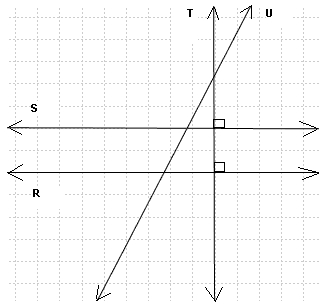# Grade 4 Geometry Questions and Problems With Solutions and Explanations

Solutions and explanations to Grade 4 geometry problems are presented.

 True or False: Two parallel lines intersect. Solution False: Two parallel lines do not intersect. A triangle is a closed planar shape with Solution 3 sides. (tri in the word triangle means three, from Latin) A closed planar shape with 5 sides is called a Solution pentagon. (penta in the word pentagon means five, from Greek) A closed planar shape with 4 sides is called a Solution quadrilateral. (quadri in the word quadrilateral means four, from Latin) A line segment is defined by Solution 2 points An equilateral triangle has Solution all three sides equal and all three angles equal. Which of the statements below best describes a square? Solution A square has 4 equal sides and 4 right angles. Which pair of lines are parallel?Solution The pair of lines in A are parallel because they do not intersect. Which of these shapes are quadrilaterals? triangles? squares?Solution i) quadrilaterals have 4 sides: shapes B, E F. ii) triangles have 3 sides: shapes A, D. iii) squares have 4 equal sides and 4 right angles: shape F. Which of these shapes are right triangles? rectangles?Solution i) right triangles have 1 right angle: shape B. ii) rectangles have 2 pairs of parallel sides and 4 right angles: shapes D and E. Which pair of lines are parallel? perpendicular?Solution i) parallel lines do not intersect. Lines S and R are parallel ii) perpendicular lines intersect and form a right angle (90 degrees). T and S , and T and R are pairs of perpendicular lines. Find the perimeter and area of each shape.Solution Shape A Perimeter = 5 + 5 + 5 + 5 = 20 Area = 5 � 5 = 25 Shape B Perimeter = 9 + 6 + 9 + 6 = 30 Area = 9 � 6 = 54 Shape C Perimeter = 10 + 2 + 10 + 2 = 24 Area = 10 � 2 = 20 Shape D Perimeter = 5 + 4 + 5 + 4 = 18 Area = 5 � 4 = 20 Find the perimeter and area of each shape.Solution Shape A: Perimeter is obtained by adding all sides. Starting from the top side we have Perimeter = 4 + 4 + 4 + 4 + 8 + 8 = 32 Shape A: The area of the given shape is equal to the area of the square of side 8 from which the area of the small square top right of side 4 is subtracted. Area = 8 � 8 - 4 � 4 = 64 - 16 = 48 Shape B: Perimeter is obtained by adding all sides. Starting from the top side we have Perimeter = 8 + 2 + 4 + 4 + 4 + 2 + 8 + 8 = 40 Shape B: The area of the given shape is equal to the area of the square (left side) of side 8 to which the area of the small square (right side) of side 4 is added. Area = 8 � 8 + 4 � 4 = 64 + 16 = 80 Which of these is an isosceles triangle?Solution An isosceles triangle has two equal sides: shape B Which pair of triangles are congruent?Solution Congruent shapes have sides of equal length: shapes A and C Point O is the center of the circle below. What do you call the segments AB? CD? OD?Solution A segment whose endpoints are on a circle is called a chord: AB is a chord A chord that passes through the center of circle is called a diameter: CD is a diameter A segment whose 2 endpoints are: 1) the center of circle and 2) one point on the circle is called the radius: OD is a radius Primary Maths (grades 4 and 5) with Free Questions and Problems With Answers Middle School Maths (grades 6,7,8 and 9) with Free Questions and Problems With Answers High School Maths (Grades 10, 11 and 12) - Free Questions and Problems With Answers Home Page